# phased.ULA

Uniform linear array

## Description

`phased.ULA` creates a uniform linear array (ULA) System object™ and computes its response.

To compute the response for each element in the array for specified directions:

1. Create the `phased.ULA` object and set its properties.

2. Call the object with arguments, as if it were a function.

## Creation

### Syntax

``array = phased.ULA``
``array = phased.ULA(Name=Value)``
``array = phased.ULA(N,D,Name=Value)``

### Description

````array = phased.ULA` creates a uniform linear `array` (ULA) System object. In this syntax, the object models a ULA formed with identical isotropic phased array sensor elements. The origin of the local coordinate system is the phase center of the array. The positive x-axis is the direction normal to the array, and the elements of the array are located along the y-axis.```
````array = phased.ULA(Name=Value)` creates the object `array` with each specified property `Name` set to the specified `Value`. You can specify additional name-value pair arguments in any order as (`Name1` = `Value1`, … ,`NameN` = `ValueN`).```

example

````array = phased.ULA(N,D,Name=Value)` creates a ULA `array` object with the `NumElements` property set to `N`, the `ElementSpacing` property set to `D`, and other specified property Names set to the specified Values. `N` and `D` are value-only arguments. When specifying a value-only argument, specify all preceding value-only arguments. You can specify name-value pair arguments in any order.```

## Properties

expand all

Unless otherwise indicated, properties are nontunable, which means you cannot change their values after calling the object. Objects lock when you call them, and the `release` function unlocks them.

If a property is tunable, you can change its value at any time.

Phased array element, specified as a Phased Array System Toolbox antenna, microphone, or transducer element or Antenna Toolbox antenna.

Example: `phased.CosineAntennaElement`

Number of array elements, specified as a positive integer.

Data Types: `double`

Spacing between two adjacent elements in the array, specified as a positive scalar. Units are in meters.

Data Types: `double`

Axis of linear array, specified as `'y'`, `'x'`, or `'z'`. Linear array elements are located along the selected coordinate system axis. The array axis determines the direction along which the element normal vectors point.

ArrayAxis Property ValueElement Normal Direction
`'x'`azimuth = 90°, elevation = 0° (y-axis)
`'y'` (default)azimuth = 0°, elevation = 0° (x-axis)
`'z'`azimuth = 0°, elevation = 0° (x-axis)

Example: `'z'`

Data Types: `char` | `string`

Array element tapering, specified as a complex-valued scalar, 1-by-N complex-valued row vector, or N-by-1 complex-valued column vector. N represents the number of elements of the array. Tapers, also known as weights, are applied to each sensor element in the sensor array and modify both the amplitude and phase of the received data. If `'Taper'` is a scalar, the same taper value is applied to all elements. If `'Taper'` is a vector, each taper value is applied to the corresponding sensor element.

Data Types: `double`

## Usage

### Syntax

``resp = array(freq,ang)``

### Description

example

````resp = array(freq,ang)` returns the array element responses, `resp`, at the operating frequencies specified in `freq` and in directions specified in `ang`.```

### Input Arguments

expand all

Array operating frequencies, specified as a length-L row vector. Typical values are within the range specified by a frequency range property of the array element. That property is called `FrequencyRange` or `FrequencyVector`, depending on the type of array element. The element has zero response at frequencies outside that range. Units are in Hz.

Data Types: `double`

Array response directions, specified as a 2-by-M matrix or length-M row vector.

If `ANG` is a 2-by-M matrix, each column of the matrix specifies the direction in the form ```[azimuth; elevation]```. The azimuth angle must lie between –180° and 180°, inclusive. The elevation angle must lie between –90° and 90°, inclusive.

If `ang` is a row vector of length M, each element specifies the azimuth angle of the direction. In this case, the corresponding elevation angle is assumed to be 0°.

Units are in degrees.

### Output Arguments

expand all

Voltage responses of the phased array. The output depends on whether the array supports polarization or not.

• If the array is not capable of supporting polarization, the voltage response, `resp`, has the dimensions N-by-M-by-L. N is the number of elements in the array. The dimension M is the number of angles specified in `ang`. L is the number of frequencies specified in `freq`. For any element, the columns of `resp` contain the responses of the array elements for the corresponding direction specified in `ang`. Each of the L pages of `resp` contains the responses of the array elements for the corresponding frequency specified in `freq`.

• If the array is capable of supporting polarization, the voltage response, `resp`, is a MATLAB `struct` containing two fields, `resp.H` and `resp.V`. The field, `resp.H`, represents the array’s horizontal polarization response, while `resp.V` represents the array’s vertical polarization response. Each field has the dimensions N-by-M-by-L. N is the number of elements in the array, and M is the number of angles specified in `ang`. L is the number of frequencies specified in `freq`. Each column of `resp` contains the responses of the array elements for the corresponding direction specified in `ang`. Each of the L pages of `ang` contains the responses of the array elements for the corresponding frequency specified in `freq`.

## Object Functions

To use an object function, specify the System object as the first input argument. For example, to release system resources of a System object named `obj`, use this syntax:

`release(obj)`

expand all

 `beamwidth` Compute and display beamwidth of an array `collectPlaneWave` Simulate received plane waves at array `directivity` Compute array directivity `getElementNormal` Normal vectors for array elements `getElementPosition` Positions of array elements `getNumElements` Number of elements in an array `getTaper` Array element tapers `isPolarizationCapable` Array polarization capability `pattern` Plot array directivity and patterns `patternAzimuth` Plot array directivity or pattern versus azimuth `patternElevation` Plot array directivity or pattern versus elevation `perturbations` Perturbations defined on array `perturbedArray` Apply perturbations to phased array `perturbedPattern` Display pattern of perturbed array `plotGratingLobeDiagram` Plot grating lobe diagram of array `thinnedArray` Create thinned array from full array `viewArray` View array geometry
 `step` Run System object algorithm `release` Release resources and allow changes to System object property values and input characteristics `reset` Reset internal states of System object

## Examples

collapse all

Compute the directivities of two different uniform linear arrays (ULA). One array consists of isotropic antenna elements and the second array consists of cosine antenna elements. In addition, compute the directivity when the first array is steered in a specified direction. For each case, calculate the directivities for a set of seven different azimuth directions all at zero degrees elevation. Set the frequency to 300 MHz.

Array of isotropic antenna elements

First, create a 10-element ULA of isotropic antenna elements spaced 1/2-wavelength apart.

```c = physconst('LightSpeed'); fc = 300e6; lambda = c/fc; ang = [-30,-20,-10,0,10,20,30; 0,0,0,0,0,0,0]; myAnt1 = phased.IsotropicAntennaElement; myArray1 = phased.ULA(10,lambda/2,'Element',myAnt1);```

Compute the directivity.

`d = directivity(myArray1,fc,ang,'PropagationSpeed',c)`
```d = 7×1 -6.9886 -6.2283 -6.5176 10.0011 -6.5176 -6.2283 -6.9886 ```

Array of cosine antenna elements

Next, create a 10-element ULA of cosine antenna elements spaced 1/2-wavelength apart.

```myAnt2 = phased.CosineAntennaElement('CosinePower',[1.8,1.8]); myArray2 = phased.ULA(10,lambda/2,'Element',myAnt2);```

Compute the directivity.

`d = directivity(myArray2,fc,ang,'PropagationSpeed',c)`
```d = 7×1 -1.9838 0.0529 0.4968 17.2548 0.4968 0.0529 -1.9838 ```

The directivity of the cosine ULA is greater than the directivity of the isotropic ULA because of the larger directivity of the cosine antenna element.

Steered array of isotropic antenna elements

Finally, steer the isotropic antenna array to 30 degrees in azimuth and compute the directivity.

```w = steervec(getElementPosition(myArray1)/lambda,[30;0]); d = directivity(myArray1,fc,ang,'PropagationSpeed',c,... 'Weights',w)```
```d = 7×1 -297.5224 -13.9783 -9.5713 -6.9897 -4.5787 -2.0536 10.0000 ```

The directivity is greatest in the steered direction.

Construct three ULAs with elements along the x-, y-, and z-axes. Obtain the element normals.

First, choose the array axis along the x-axis.

```sULA1 = phased.ULA('NumElements',5,'ArrayAxis','x'); norm = getElementNormal(sULA1)```
```norm = 2×5 90 90 90 90 90 0 0 0 0 0 ```

The element normal vectors point along the y-axis.

Next, choose the array axis along the y-axis.

```sULA2 = phased.ULA('NumElements',5,'ArrayAxis','y'); norm = getElementNormal(sULA2)```
```norm = 2×5 0 0 0 0 0 0 0 0 0 0 ```

The element normal vectors point along the x-axis.

Finally, set the array axis along the z-axis. Obtain the normal vectors of the odd-numbered elements.

```sULA3 = phased.ULA('NumElements',5,'ArrayAxis','z'); norm = getElementNormal(sULA3,[1,3,5])```
```norm = 2×3 0 0 0 0 0 0 ```

The element normal vectors also point along the x-axis.

Construct a ULA with 5 elements along the z-axis. Obtain the element positions.

```sULA = phased.ULA('NumElements',5,'ArrayAxis','z'); pos = getElementPosition(sULA)```
```pos = 3×5 0 0 0 0 0 0 0 0 0 0 -1.0000 -0.5000 0 0.5000 1.0000 ```

Construct a default ULA and obtain the number of elements in that array.

``` array = phased.ULA; N = getNumElements(array)```
```N = 2 ```

Draw a 6-element ULA and use the `'ShowIndex'` parameter to show the indices of the first and third elements.

```array = phased.ULA(6); viewArray(array,'ShowIndex',[1 3],'ShowNormals',true, ... 'ShowLocalCoordinates',true,'Orientation',[60;100;45], ... 'ShowAnnotation',true)```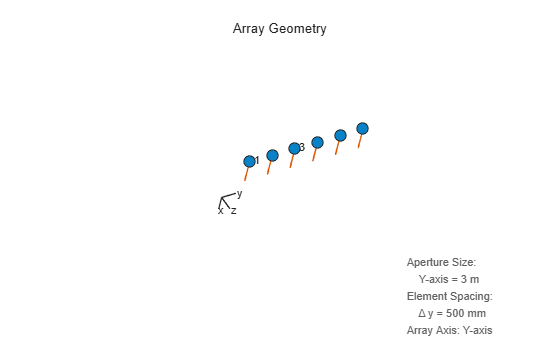Construct a 5-element ULA with a Taylor window taper. Then, obtain the element taper values.

```taper = taylorwin(5)'; array = phased.ULA(5,'Taper',taper); w = getTaper(array)```
```w = 5×1 0.5181 1.2029 1.5581 1.2029 0.5181 ```

Show that an array of `phased.ShortDipoleAntennaElement` antenna elements supports polarization.

```antenna = phased.ShortDipoleAntennaElement(... 'FrequencyRange',[1e9 10e9]); array = phased.ULA('NumElements',3,'Element',antenna); isPolarizationCapable(array)```
```ans = logical 1 ```

The returned value of `1` shows that this array supports polarization.

Create a 4-element undersampled ULA and find the response of each element at boresight. Plot the array pattern at 1 GHz for azimuth angles between -180 and 180 degrees. The default element spacing is 0.5 meters.

```array = phased.ULA('NumElements',4); fc = 1e9; ang = [0;0]; resp = array(fc,ang)```
```resp = 4×1 1 1 1 1 ```
```c = physconst('LightSpeed'); pattern(array,fc,-180:180,0,'PropagationSpeed',c,... 'CoordinateSystem','rectangular',... 'Type','powerdb','Normalize',true)```Construct a 10-element uniform linear array of omnidirectional microphones spaced 3 cm apart. Then, plot the array pattern at 100 Hz.

```mic = phased.OmnidirectionalMicrophoneElement(... 'FrequencyRange',[20 20e3]); Nele = 10; array = phased.ULA('NumElements',Nele,... 'ElementSpacing',3e-2,... 'Element',mic); fc = 100; ang = [0; 0]; resp = array(fc,ang); c = 340; pattern(array,fc,[-180:180],0,'PropagationSpeed',c,... 'CoordinateSystem','polar',... 'Type','powerdb',... 'Normalize',true);```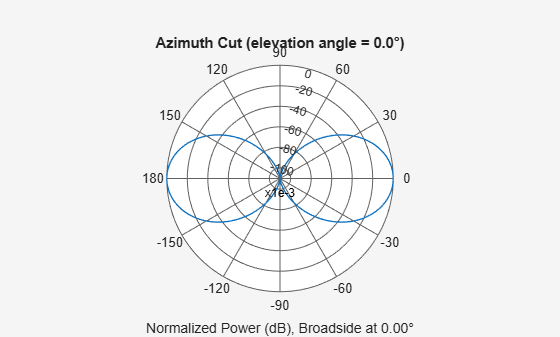Build a tapered uniform line array of 5 short-dipole sensor elements. Because short dipoles support polarization, the array should as well. Verify that it supports polarization by looking at the output of the `isPolarizationCapable` method.

```antenna = phased.ShortDipoleAntennaElement(... 'FrequencyRange',[100e6 1e9],'AxisDirection','Z'); array = phased.ULA('NumElements',5,'Element',antenna,... 'Taper',[.5,.7,1,.7,.5]); isPolarizationCapable(array)```
```ans = logical 1 ```

Then, draw the array using the `viewArray` method.

`viewArray(array,'ShowTaper',true,'ShowIndex','All')`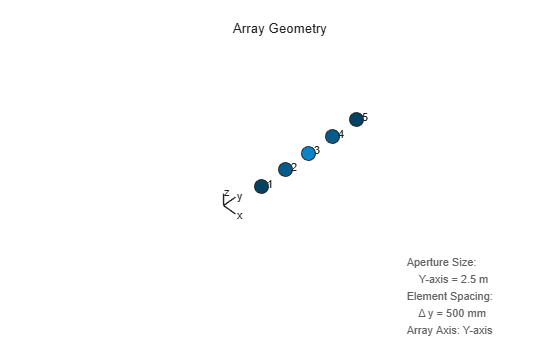Compute the horizontal and vertical responses.

```fc = 150e6; ang = ; resp = array(fc,ang);```

Display the horizontal polarization response.

`resp.H`
```ans = 5×1 0 0 0 0 0 ```

Display the vertical polarization response.

`resp.V`
```ans = 5×1 -0.6124 -0.8573 -1.2247 -0.8573 -0.6124 ```

Plot an azimuth cut of the vertical polarization response.

```c = physconst('LightSpeed'); pattern(array,fc,[-180:180],0,... 'PropagationSpeed',c,... 'CoordinateSystem','polar',... 'Polarization','V',... 'Type','powerdb',... 'Normalize',true)```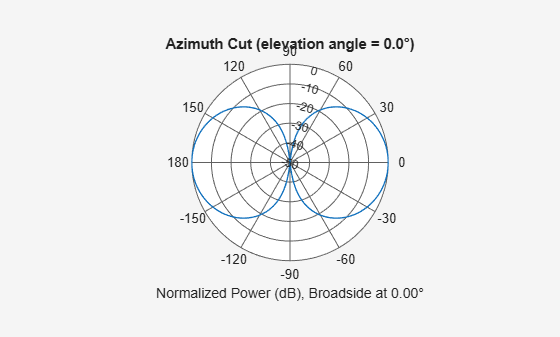Create an 9-element ULA of short dipole antenna elements spaced 1.5 meters apart. Display the azimuth and elevation directivities. The operating frequency is 500 MHz. Plot the directivities in polar coordinates.

Evaluate the fields at 45 degrees azimuth and 0 degrees elevation.

```element = phased.ShortDipoleAntennaElement(... 'FrequencyRange',[50e6,1000e6],... 'AxisDirection','Z'); array = phased.ULA('NumElements',9,'ElementSpacing',1.5,'Element',element); fc = 500e6; ang = [45;0]; resp = array(fc,ang); disp(resp.V)```
``` -1.2247 -1.2247 -1.2247 -1.2247 -1.2247 -1.2247 -1.2247 -1.2247 -1.2247 ```

Display the azimuth directivity pattern at 500 MHz for azimuth angles between -180 and 180 degrees.

```c = physconst('LightSpeed'); pattern(array,fc,[-180:180],0,... 'Type','directivity',... 'PropagationSpeed',c)```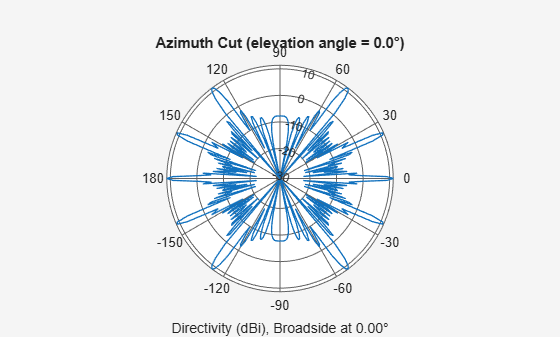Display the elevation directivity pattern at 500 MHz for elevation angles between -90 and 90 degrees.

```pattern(array,fc,,[-90:90],... 'Type','directivity',... 'PropagationSpeed',c)```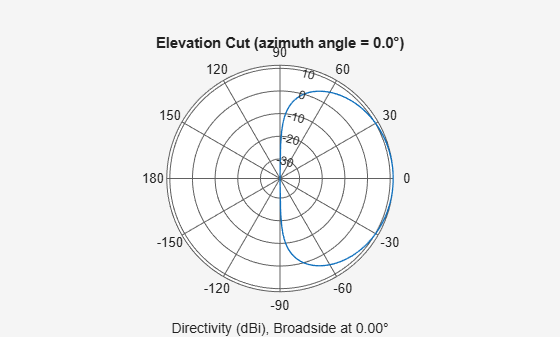Create a 10-element ULA antenna array consisting of cosine antenna elements spaced 10 cm apart. Display the 3-D power pattern in UV space. The operating frequency is 500 MHz.

```sCos = phased.CosineAntennaElement('FrequencyRange',[100e6 1e9],... 'CosinePower',[2.5,2.5]); sULA = phased.ULA('NumElements',10,... 'ElementSpacing',.1,... 'Element',sCos); c = physconst('LightSpeed'); fc = 500e6; pattern(sULA,fc,[-1:.01:1],[-1:.01:1],... 'CoordinateSystem','uv',... 'Type','powerdb',... 'PropagationSpeed',c)```Create a 7-element ULA of short-dipole antenna elements spaced 10 cm apart. Plot an azimuth cut of directivity at 0 and 10 degrees elevation. Assume the operating frequency is 500 MHz.

```fc = 500e6; sCDant = phased.ShortDipoleAntennaElement('FrequencyRange',[100,900]*1e6); sULA = phased.ULA('NumElements',7,'ElementSpacing',0.1,'Element',sCDant); patternAzimuth(sULA,fc,[0 30])```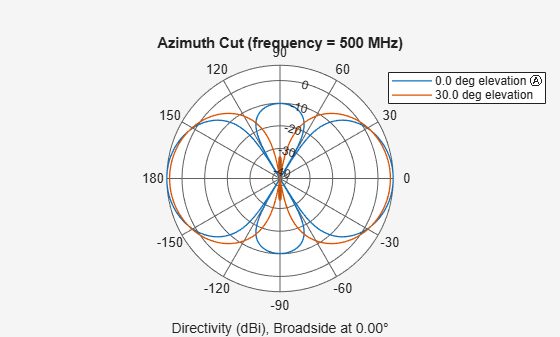You can plot a smaller range of azimuth angles by setting the `Azimuth` property.

`patternAzimuth(sULA,fc,[0 30],'Azimuth',[-90:90])`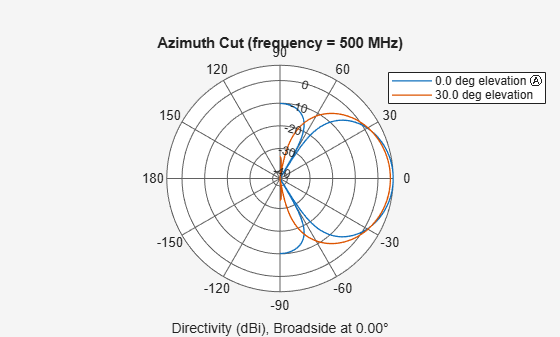Create a 6-element ULA of short-dipole antenna elements with element spacing of 10 cm. Plot an elevation cut of directivity at 0 and 90 degrees azimuth. Assume the operating frequency is 500 MHz.

```fc = 500e6; c = physconst('LightSpeed'); elem = phased.ShortDipoleAntennaElement('FrequencyRange',[100,900]*1e6); array= phased.ULA('NumElements',6,'ElementSpacing',0.1,'Element',elem); patternElevation(array,fc,[0 90],'PropagationSpeed',c) legend('Location','southwest')```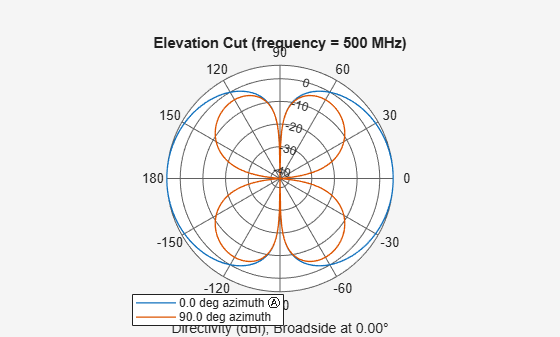You can plot a smaller range of elevation angles by setting the `Elevation` property to [0:90] degrees but now with cuts at 0 and 45 degrees azimuth.

```patternElevation(array,fc,[0 45],'Elevation',[0:90],'PropagationSpeed',c) legend('Location','southwest')```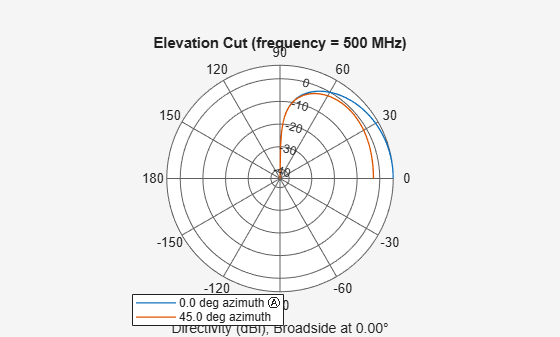Plot the beamwidth of a sonar array operating at a frequency of 2 kHz when the propagation speed of sound in water is 1500 m/s.

The sonar array consists of a 20-element uniform linear array (ULA). Consider the element of the ULA to be a backbaffled `phased.IsotropicProjector` with a `VoltageResponse` of 100 Volts and with a `FrequencyRange` from 10 Hz to 300 kHz. Create a `phased.ULA` object to model the uniform linear array.

``` projector = phased.IsotropicProjector('BackBaffled',true,... 'VoltageResponse',100,'FrequencyRange',[10 300000])```
```projector = phased.IsotropicProjector with properties: VoltageResponse: 100 FrequencyRange: [10 300000] BackBaffled: true ```
```myArray = phased.ULA('Element',projector,'NumElements',20,... 'ElementSpacing',1500/200e3/2)```
```myArray = phased.ULA with properties: Element: [1x1 phased.IsotropicProjector] NumElements: 20 ElementSpacing: 0.0037 ArrayAxis: 'y' Taper: 1 ```

Using the `beamwidth` function, calculate and plot the 6 dB beamwidth of the sonar array.

`beamwidth(myArray,200e3,'dBDown',6,'PropagationSpeed',1500)`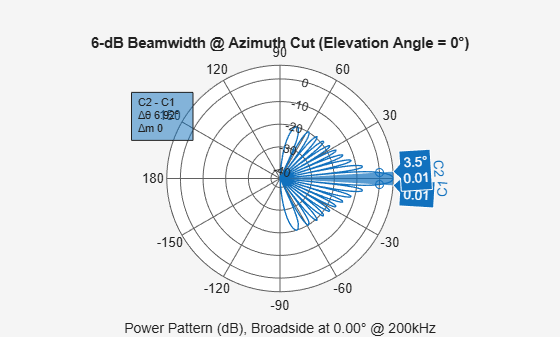```ans = 6.9200 ```

Calculate the half-power beamwidth and angles of a 20-element uniform linear array (ULA) of cosine antenna elements.

Create a `phased.CosineAntennaElement` object with the `'CosinePower'` exponents set to 1.5.

`myAnt = phased.CosineAntennaElement`
```myAnt = phased.CosineAntennaElement with properties: FrequencyRange: [0 1.0000e+20] CosinePower: [1.5000 1.5000] ```

Create a `phased.ULA` object to model a 20-element ULA of cosine antenna elements. These elements are spaced at 0.5 meters on the azimuth plane.

`array = phased.ULA('Element',myAnt,'NumElements',20)`
```array = phased.ULA with properties: Element: [1x1 phased.CosineAntennaElement] NumElements: 20 ElementSpacing: 0.5000 ArrayAxis: 'y' Taper: 1 ```

Compute the beamwidth and angles of the array when it is operating at 3e8 Hz. Specify the beamwidth to be computed along the elevation plane.

`[BW,Ang] = beamwidth(array,3e8,'Cut','Elevation')`
```BW = 74.8200 ```
```Ang = 1×2 -37.4100 37.4100 ```

Create a 4-element ULA of isotropic antenna elements and find the response of each element at boresight. Plot the array response at 1 GHz for azimuth angles between -180 and 180 degrees.

```ha = phased.ULA('NumElements',4); fc = 1e9; ang = [0;0]; resp = step(ha,fc,ang); c = physconst('LightSpeed'); pattern(ha,fc,[-180:180],0,... 'PropagationSpeed',c,... 'CoordinateSystem','rectangular')```Find the response of a ULA array of 10 omnidirectional microphones spaced 1.5 meters apart. Set the frequency response of the microphone to the range 20 Hz to 20 kHz and choose the signal frequency to be 100 Hz. Using the `step` method, determine the response of each element at boresight: 0 degrees azimuth and 0 degrees elevation.

```mic = phased.OmnidirectionalMicrophoneElement( ... 'FrequencyRange',[20 20e3]); Nelem = 10; array = phased.ULA('NumElements',Nelem, ... 'ElementSpacing',1.5,'Element',mic); fc = 100; ang = [0;0]; resp = array(fc,ang)```
```resp = 10×1 1 1 1 1 1 1 1 1 1 1 ```

Plot the array directivity. Assume the speed of sound in air to be 340 m/s.

```c = 340; pattern(array,fc,[-180:180],0,'PropagationSpeed',c,'CoordinateSystem','polar')```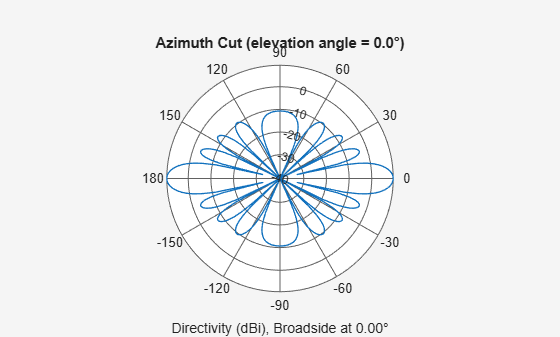Simulate two received plane-wave random signals at a 4-element ULA. The signals arrive from 10° and 30° azimuth. Both signals have an elevation angle of 0°. Assume the propagation speed is the speed of light and the carrier frequency of the signal is 100 MHz.

```array = phased.ULA(4); y = collectPlaneWave(array,randn(4,2),[10 30],100e6,physconst('LightSpeed'))```
```y = 4×4 complex 0.7430 - 0.3705i 0.8433 - 0.1314i 0.8433 + 0.1314i 0.7430 + 0.3705i 0.8418 + 0.4308i 0.5632 + 0.1721i 0.5632 - 0.1721i 0.8418 - 0.4308i -2.4817 + 0.9157i -2.6683 + 0.3175i -2.6683 - 0.3175i -2.4817 - 0.9157i 1.0724 - 0.4748i 1.1895 - 0.1671i 1.1895 + 0.1671i 1.0724 + 0.4748i ```

Plot the grating lobe diagram for a 4-element uniform linear array having element spacing less than one-half wavelength. Grating lobes are plotted in u-v coordinates.

Assume the operating frequency of the array is 3 GHz and the spacing between elements is 0.45 of the wavelength. All elements are isotropic antenna elements. Steer the array in the direction 45 degrees in azimuth and 0 degrees in elevation.

```c = physconst('LightSpeed'); f = 3e9; lambda = c/f; sIso = phased.IsotropicAntennaElement; sULA = phased.ULA('Element',sIso,'NumElements',4,... 'ElementSpacing',0.45*lambda); plotGratingLobeDiagram(sULA,f,[45;0],c);```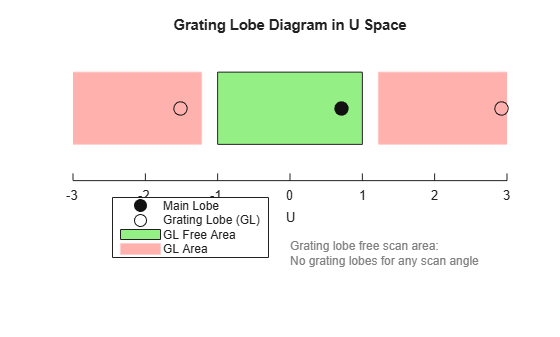The main lobe of the array is indicated by a filled black circle. The grating lobes in the visible and nonvisible regions are indicated by empty black circles. The visible region is defined by the direction cosine limits between [-1,1] and is marked by the two vertical black lines. Because the array spacing is less than one-half wavelength, there are no grating lobes in the visible region of space. There are an infinite number of grating lobes in the nonvisible regions, but only those in the range [-3,3] are shown.

The grating-lobe free region, shown in green, is the range of directions of the main lobe for which there are no grating lobes in the visible region. In this case, it coincides with the visible region.

The white area of the diagram indicates a region where no grating lobes are possible.

Plot the grating lobe diagram for a 4-element uniform linear array having element spacing greater than one-half wavelength. Grating lobes are plotted in u-v coordinates.

Assume the operating frequency of the array is 3 GHz and the spacing between elements is 0.65 of a wavelength. All elements are isotropic antenna elements. Steer the array in the direction 45 degrees in azimuth and 0 degrees in elevation.

```c = physconst('LightSpeed'); f = 3e9; lambda = c/f; sIso = phased.IsotropicAntennaElement; sULA = phased.ULA('Element',sIso,'NumElements',4,'ElementSpacing',0.65*lambda); plotGratingLobeDiagram(sULA,f,[45;0],c);```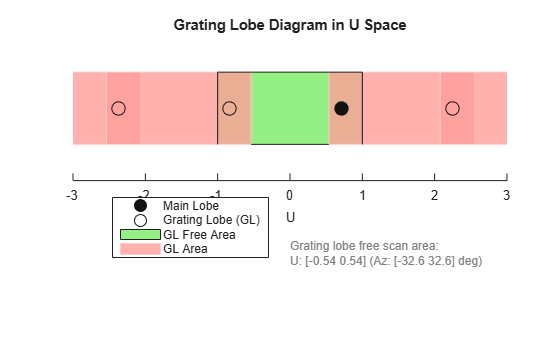The main lobe of the array is indicated by a filled black circle. The grating lobes in the visible and nonvisible regions are indicated by empty black circles. The visible region, marked by the two black vertical lines, corresponds to arrival angles between -90 and 90 degrees. The visible region is defined by the direction cosine limits $-1\le u\le 1$. Because the array spacing is greater than one-half wavelength, there is now a grating lobe in the visible region of space. There are an infinite number of grating lobes in the nonvisible regions, but only those for which $-3\le u\le 3$ are shown.

The grating-lobe free region, shown in green, is the range of directions of the main lobe for which there are no grating lobes in the visible region. In this case, it lies inside the visible region.

Plot the grating lobe diagram for a 4-element uniform linear array having element spacing greater than one-half wavelength. Apply a phase-shifter frequency that differs from the signal frequency. Grating lobes are plotted in u-v coordinates.

Assume the signal frequency is 3 GHz and the spacing between elements is 0.65 $\lambda$. All elements are isotropic antenna elements. The phase-shifter frequency is set to 3.5 GHz. Steer the array in the direction $4{5}^{\circ }$ azimuth, ${0}^{\circ }$ elevation.

```c = physconst('LightSpeed'); f = 3e9; f0 = 3.5e9; lambda = c/f; sIso = phased.IsotropicAntennaElement; sULA = phased.ULA('Element',sIso,'NumElements',4,... 'ElementSpacing',0.65*lambda ); plotGratingLobeDiagram(sULA,f,[45;0],c,f0);```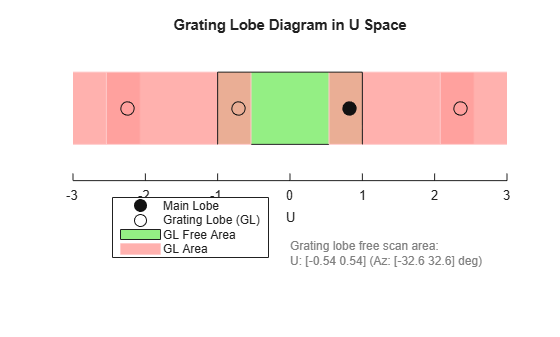As a result of adding the shifted frequency, the mainlobe shifts right towards larger $u$ values. The beam no longer points toward the actual source arrival angle.

The mainlobe of the array is indicated by a filled black circle. The grating lobes in the visible and nonvisible regions are indicated by empty black circles. The visible region, marked by the two black vertical lines, corresponds to arrival angles between ${\text{-}\text{90}}^{\circ }$ and ${\text{90}}^{\circ }$. The visible region is defined by the direction cosine limits $-1\le u\le 1$. Because the array spacing is greater than one-half wavelength, there is now a grating lobe in the visible region of space. There are an infinite number of grating lobes in the nonvisible regions, but only those for which $-3\le u\le 3$ are shown.

The grating-lobe free region, shown in green, is the range of directions of the main lobe for which there are no grating lobes in the visible region. In this case, it lies inside the visible region.

 Brookner, E., ed. Radar Technology. Lexington, MA: LexBook, 1996.

 Van Trees, H. Optimum Array Processing. New York: Wiley-Interscience, 2002.